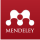# Quadrature formulas for the calculation of the Riemann-Liuville fractional integral

• Anis F. Galimyanov Kazan (Privolzhsky) Federal University
• Almaz F. Gilemzyanov Kazan (Privolzhsky) Federal University
• Chulpan B. Minnegalieva Kazan (Privolzhsky) Federal University
Keywords: Quadrature formula, fractional integration, fractional calculation, fractional Riemann-Liouville integral.

### Abstract

The quadrature formulas for the fractional Riemann-Liouville integral are investigated in this

article. A linear operator is introduced that associates ] , [ ) ( baCx  a polynomial n n P (; x)H

satisfying the condition ( )( ) ( )( ) a n j a j I P x I  x   + + = , n j , 0 = , where j x – are Chebyshev points. The integrand is approximated by an algebraic polynomial. The formula of the remainder term for the quadrature formula is derived. The quadrature formulas obtained are verified using the Wolfram Mathematica computer algebra system.

### Author Biographies

Anis F. Galimyanov, Kazan (Privolzhsky) Federal University

Kazan (Privolzhsky) Federal University

Almaz F. Gilemzyanov, Kazan (Privolzhsky) Federal University

Kazan (Privolzhsky) Federal University

Chulpan B. Minnegalieva, Kazan (Privolzhsky) Federal University

Kazan (Privolzhsky) Federal University

### References

Anastassiou, G. (2017). On left multidimensional Riemann-Liouville fractional integral // Journal of Computational Analysis and Applications, 23(2), 239-248.

Bachher, M., Sarkar, N., Lahiri, A. (2015). Fractional order thermoelastic interactions in an infinite porous material due to distributed time-dependent heat sources // Meccanica, 50 (8), 2167-2178

Baleanu D., Agarwal P., Parmar R. K., Alqurashi M. M., Salahshour S. (2017). Extension of the fractional derivative operator of the Riemann- Liouville // Journal of Nonlinear Sciences and Applications, 10 (6) 2914-2924.

Chadha, A., Pandey, D. N. (2017). Approximations of Solutions of a Neutral Fractional Integro-Differential Equation // Differential Equations and Dynamical Systems, 25 (1), 117-133.

Colinas-Armijo N., Di Paola M. (2018). Step-by-step integration for fractional operators // Communications in Nonlinear Science and Numerical Simulation. , 59, 292-305.

DEMIYEVA A.G. (2017). Custom in the system of sources of entrepreneurial law of the Russian Federation and international practice (by the example of the Eeu), Astra Salvensis, Supplement No. 10, p. 43-49.

Evans R. M., Katugampola U. N., Edwards D. A. (2017). Applications of fractional calculus in solving Abel-type integral equations: Surface-volume reaction problem // Computers & Mathematics with Applications. 73 (6), 1346- 1362.

Ezzat, M. A., Sabbah, A. S., El-Bary, A. A., Ezzat, S. M. (2015). Thermoelectric Viscoelastic Fluid with Fractional Integral and Derivative Heat Transfer // Advances in Applied Mathematics and Mechanics, 7 (4), 528-548

Ezz-Eldien S. S., Doha E. H., Bhrawy A. H., El- Kalaawy A. A., Machado J. A. T. (2018). A new operational approach for solving fractional variational problems depending on indefinite integrals // Communications in Nonlinear Science and Numerical Simulation. 57, 246-263.

Galimyanov A. F. Gilemzyanov A.F., Minnegalieva C.B. (2017). Square formulas for Weil fractional integral based on trigonometric polynom. // J. Fundam. Appl. Sci., 9(1s), 1933- 1944.

Gonzalez E. A., Petras I. (2015). Advances in Fractional Calculus: Control and Signal Processing Applications // 2015 16th International Carpathian Control Conference (Iccc). 147-152.

Kaplan, M., Bekir, A. (2016). A novel analytical method for time-fractional differential equations // Optik, 127 (20), 8209-8214

Liu, D. Y., Gibaru, O., Perruquetti, W., Laleg- Kirati, T. M. (2015). Fractional Order Differentiation by Integration and Error Analysis in Noisy Environment // Ieee Transactions on Automatic Control, 60(11) 2945-2960

Mirzaee, F., Alipour, S. (2018). Approximate solution of nonlinear quadratic integral equations of fractional order via piecewise linear functions // Journal of Computational and Applied Mathematics, 331, 217-227

Nemati, A., Yousefi, S. A. (2017). A numerical scheme for solving two-dimensional fractional optimal control problems by the Ritz method combined with fractional operational matrix // Ima Journal of Mathematical Control and Information, 34 (4), 1079-1097

Ortigueira, M. D., Machado, J. A. T. (2015). What is a fractional derivative? // Journal of Computational Physics, 293, 4-13

Pospisil, M, Skripkova, L. P. (2016). Sturm's theorems for conformable fractional differential equations // Mathematical Communications, 21 (2), 273-281

Sudsutad, W., Ntouyas, S. K., Tariboon, J. (2016). On mixed type Riemann-Liouville and Hadamard fractional integral inequalities // Journal of Computational Analysis and Applications, (21), 299-314.

Toufik, M., Atangana, A. (2017). New numerical approximation of fractional derivative with non-local and non-singular kernel: Application to chaotic models // European Physical Journal Plus, 132 (10)

Villalobos Antúnez J.V. (2015). Tecnociencia, derecho y sociedad. Pilares de una modernidad inacabada, Opción, vol. 31, núm. 76, enero-abril, pp. 5-12.

Zedan, H. A., Tantawy, S. S., Sayed, Y. M. (2017). New Solutions for System of Fractional Integro-Differential Equations and Abel's Integral Equations by Chebyshev Spectral Method // Mathematical Problems in Engineering, 13

Zeng, F. H. , Zhang, Z. Q., Karniadakis, G. E. (2017). Second-order numerical methods for multi-term fractional differential equations: Smooth and non-smooth solutions // Computer Methods in Applied Mechanics and Engineering, 327, 478-502
Published
2018-08-30
How to Cite
Galimyanov, A., Gilemzyanov, A., & Minnegalieva, C. (2018). Quadrature formulas for the calculation of the Riemann-Liuville fractional integral. Amazonia Investiga, 7(15), 74-80. Retrieved from https://amazoniainvestiga.info/index.php/amazonia/article/view/400
Issue
Section
Articles